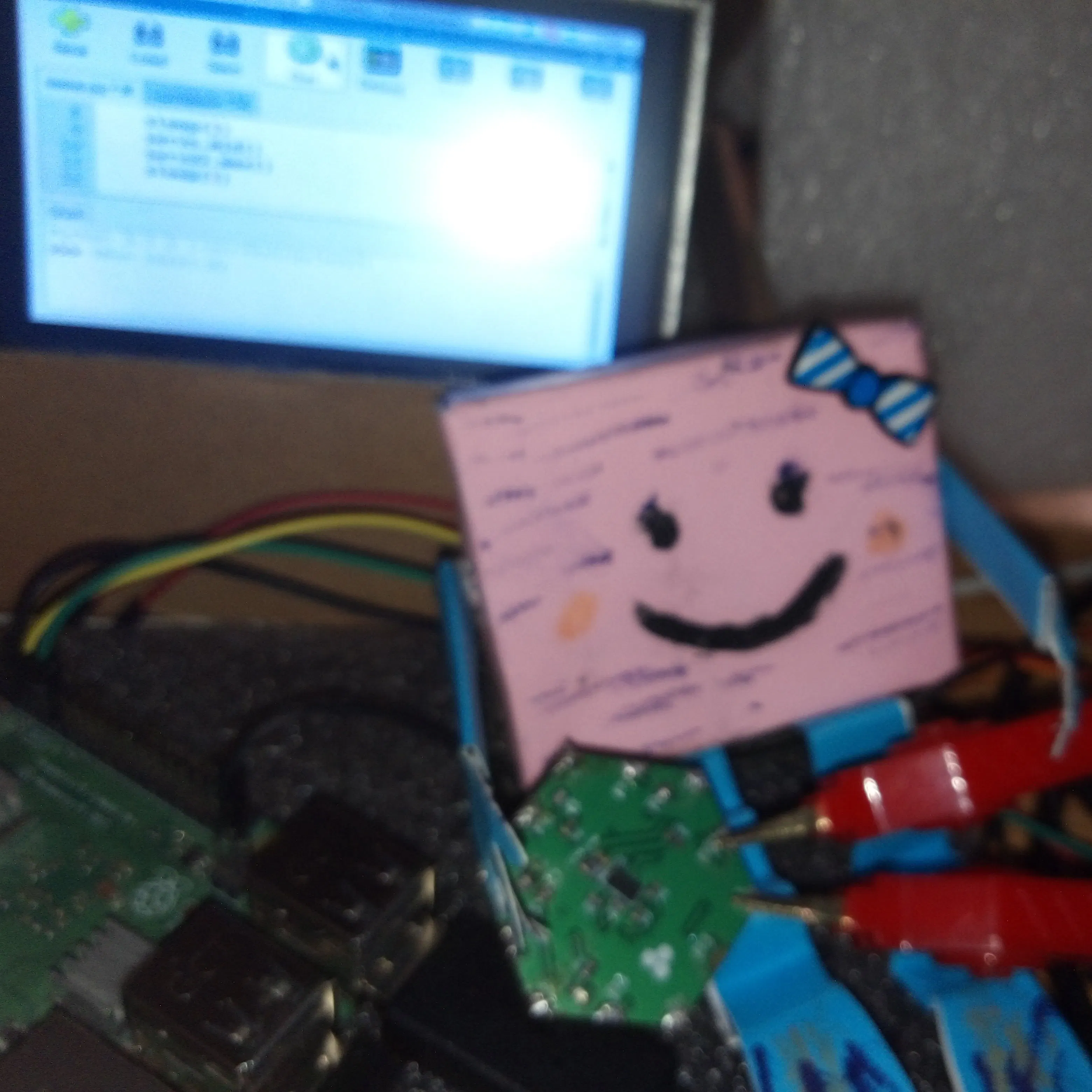# Hexabitz Piano Controllable from Raspberry Pi and Python GUI

This project uses Hexabitz modules to play the music notes. It’s definitely an effective way to learn about modules, Python GUI & music 🎶

IntermediateFull instructions provided5 hours789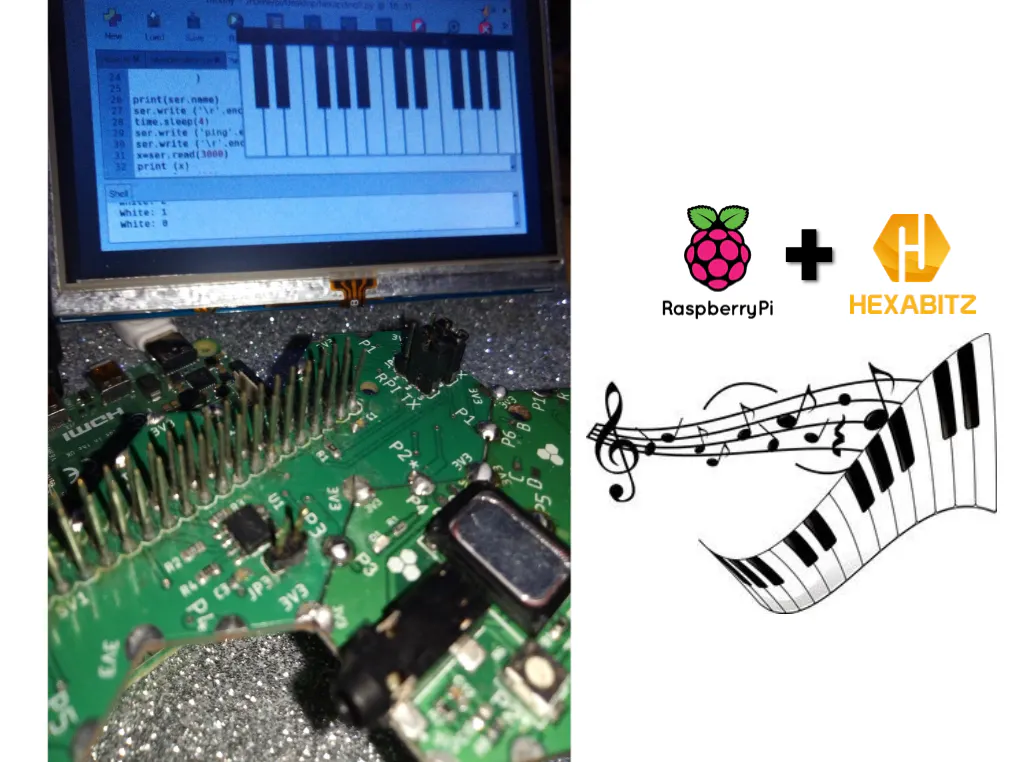## Things used in this project

### Hardware components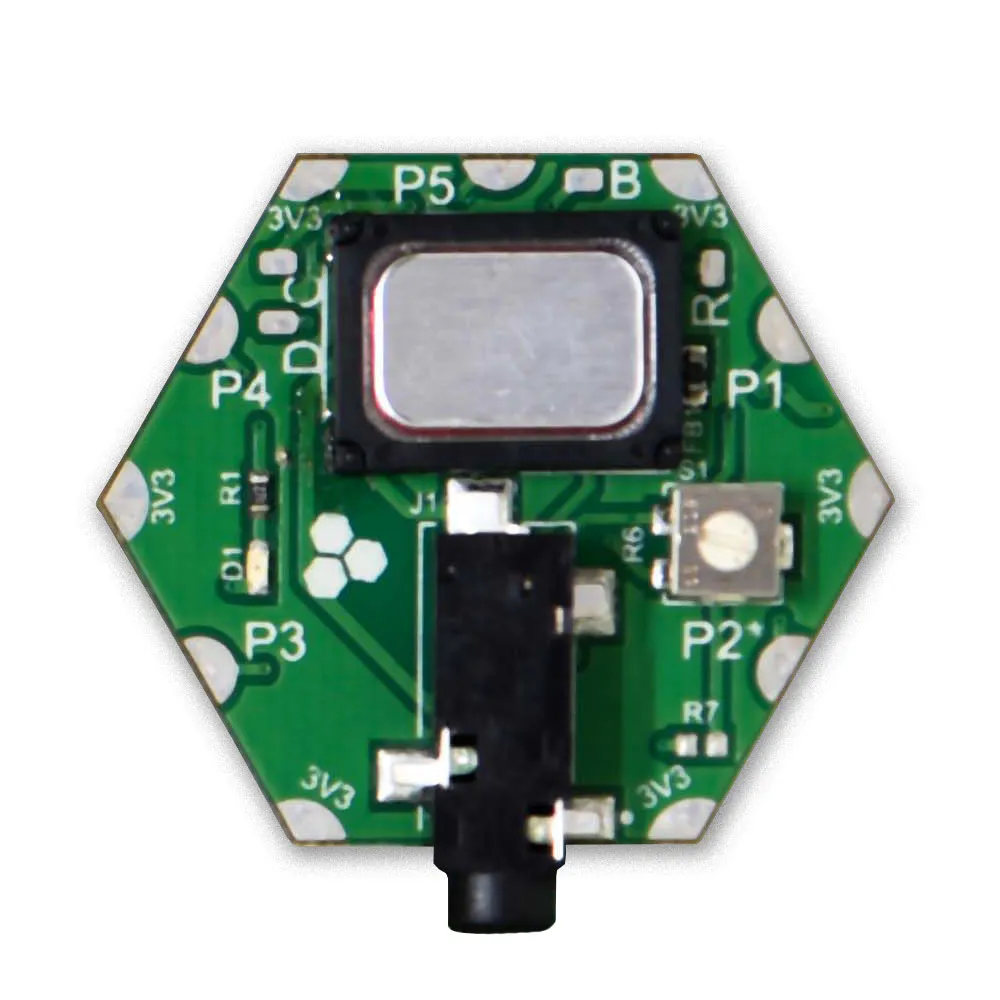Hexabitz Audio Speaker Module (H07R30)
×1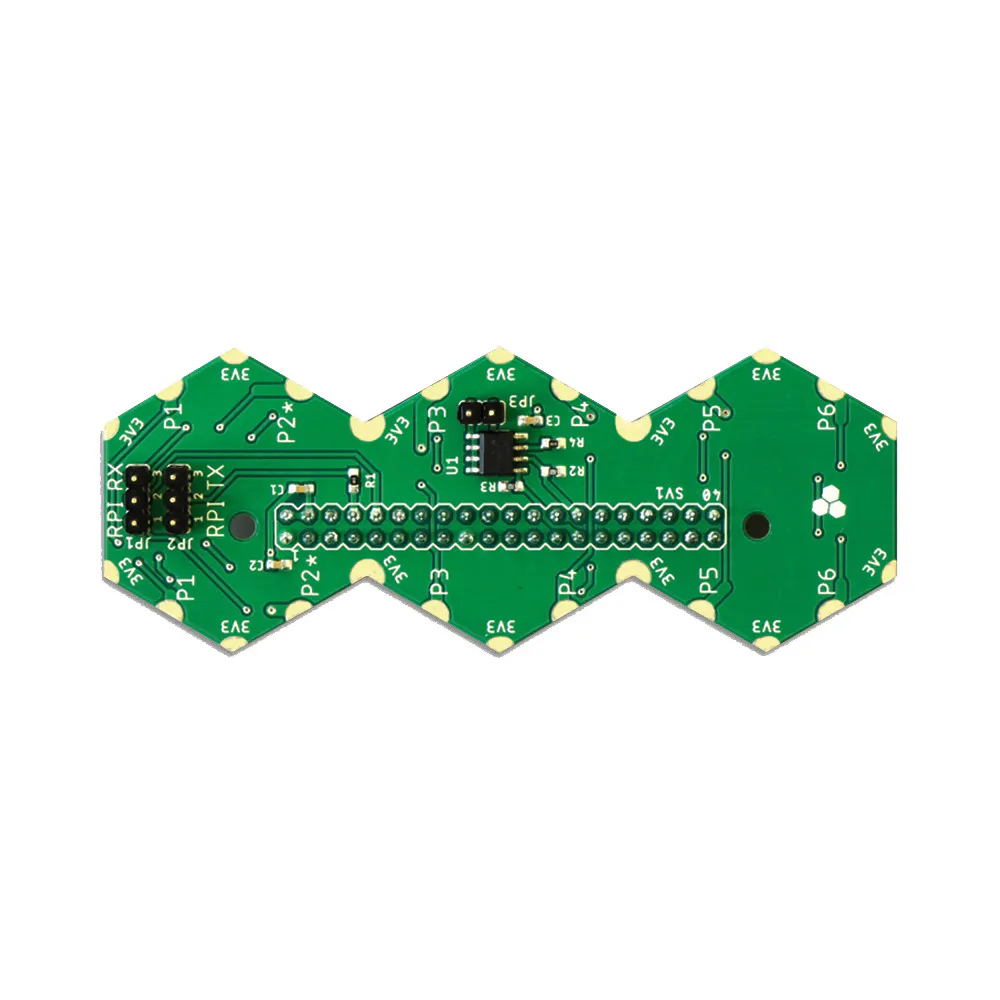Hexabitz Raspberry Pi Interface Module (HF1R0x)
×1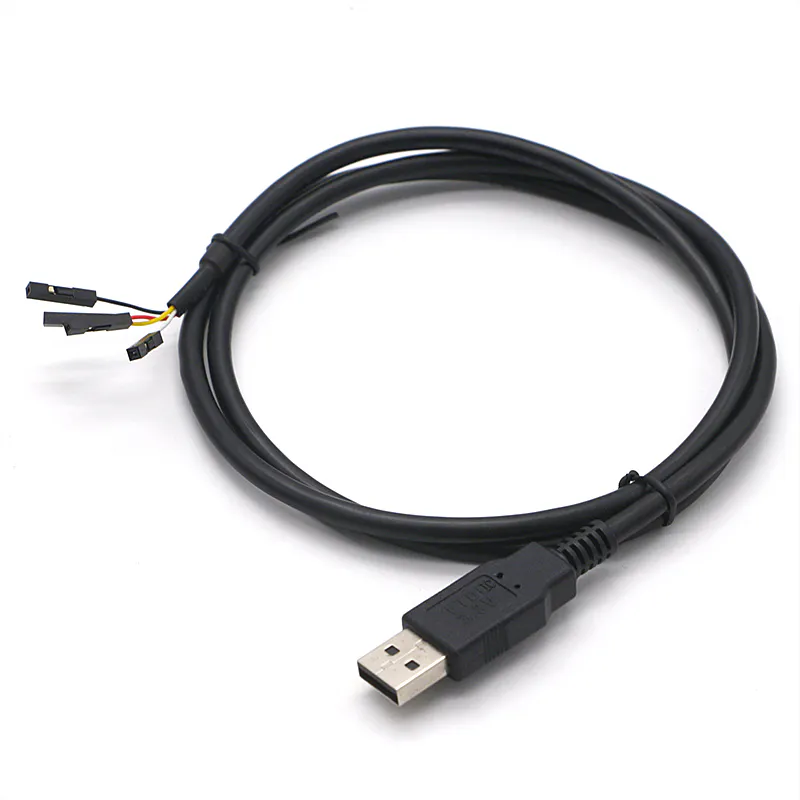Hexabitz 4-Pin USB-Serial Prototype Cable
×1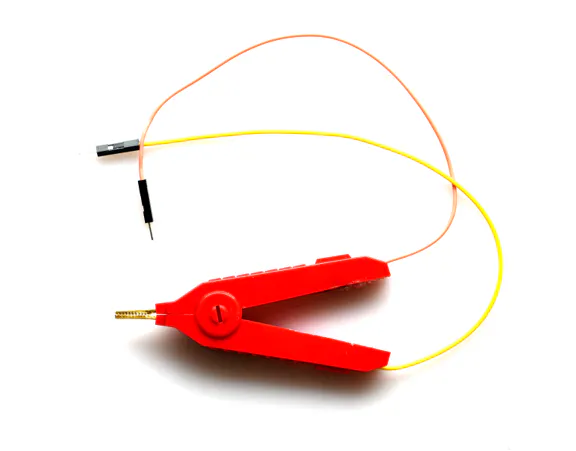Hexabitz BitzClamp
×1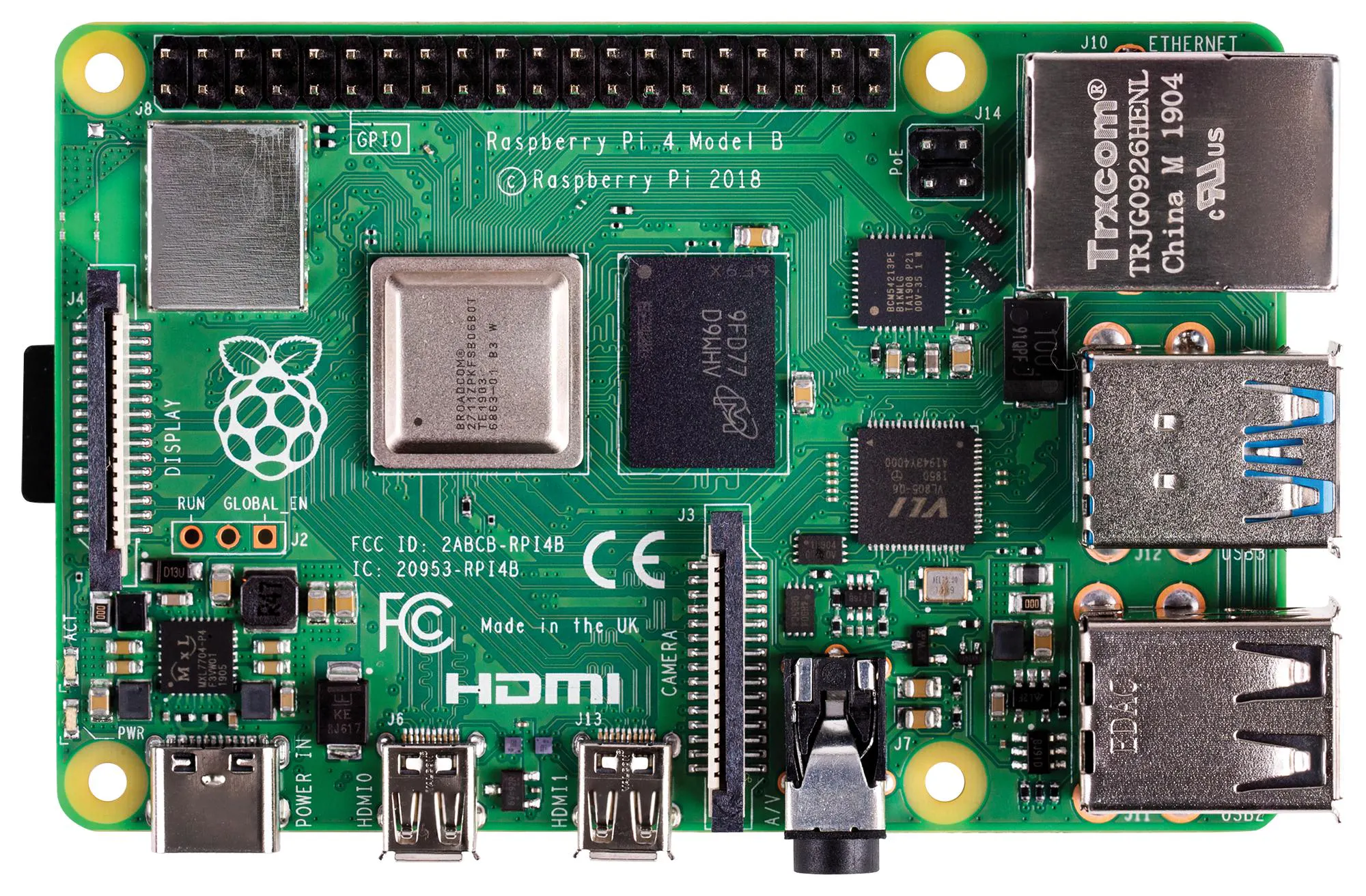Raspberry Pi 4 Model B
×1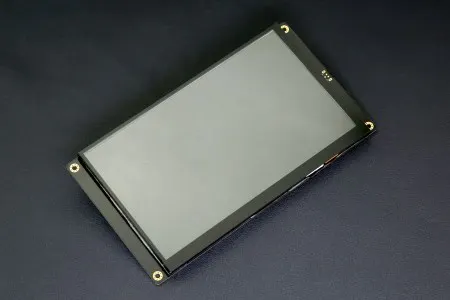DFRobot 7'' HDMI Display with Capacitive Touchscreen
×1
 Amazon Web Services Micro HDMI to HDMI Cable Adapter
×1

## Schematics

### Hexabitz Piano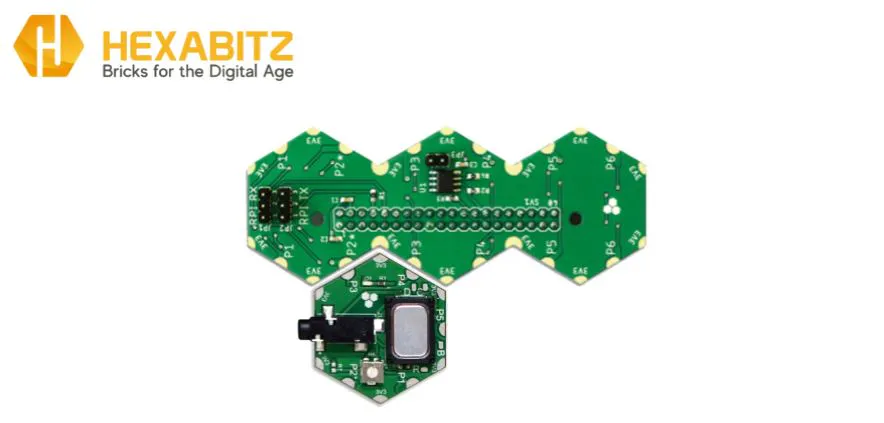## Code

### Audio Module Test

Python
```#AulaTest2020
import time
import serial
ser = serial.Serial(
port='/dev/ttyUSB0',
baudrate = 921600,
parity=serial.PARITY_NONE,
stopbits=serial.STOPBITS_ONE,
bytesize=serial.EIGHTBITS,
timeout=1
)
print(ser.name)
ser.write ('\r'.encode())
time.sleep(4)
ser.write ('demo'.encode())
ser.write ('\r'.encode())
print (x)
time.sleep(10)

ser.write ('Play tune C4 C5 D6 G7'.encode())
ser.write ('\r'.encode())
print (x1)
```

### Piano GUI Test with FTDI USB-UART 3.3V cable

Python
```# -*- coding: utf-8 -*-
"""
Created on Sat Jul 25 18:26:13 2020

@author: AulaJ
"""
import time
import serial
import tkinter as tk
ser = serial.Serial(
port='/dev/ttyUSB0',
baudrate = 921600,
parity=serial.PARITY_NONE,
stopbits=serial.STOPBITS_ONE,
bytesize=serial.EIGHTBITS,
timeout=1
)

print(ser.name)
ser.write ('\r'.encode())
time.sleep(4)
ser.write ('ping'.encode())
ser.write ('\r'.encode())
print (x)
time.sleep(10)
def clicked(color, num):
print(color + ': ' + str(num))
if(num ==0):
ser.write ('Play tune c7)'.encode())
ser.write ('\r'.encode())
elif(num ==1):
ser.write ('Play tune d6'.encode())
ser.write ('\r'.encode())
elif(num ==2):
ser.write ('Play tune e6'.encode())
ser.write ('\r'.encode())

elif(num ==3):
ser.write ('Play tune f6'.encode())
ser.write ('\r'.encode())
elif(num ==4):
ser.write ('Play tune G6'.encode())
ser.write ('\r'.encode())
elif(num ==5):
ser.write ('Play tune a5'.encode())
ser.write ('\r'.encode())
elif(num ==6):
ser.write ('Play tune b6'.encode())
ser.write ('\r'.encode())
elif(num ==7):
ser.write ('Play tune c5'.encode())
ser.write ('\r'.encode())
elif(num ==8):
ser.write ('Play tune d8'.encode())
ser.write ('\r'.encode())
elif(num ==9):
ser.write ('Play tune e7'.encode())
ser.write ('\r'.encode())

root = tk.Tk()

scales = 3

root.geometry('{}x200'.format(300 * scales))

white_keys = 7 * scales
black = [1, 1, 0, 1, 1, 1, 0] * scales

for i in range(white_keys):
tk.Button(root, bg='white', activebackground='gray87', command=lambda i=i: clicked('White', i)).grid(row=0, column=i*3, rowspan=2, columnspan=3, sticky='nsew')

for i in range(white_keys - 1):
if black[i]:
tk.Button(root, bg='black', activebackground='gray12', command=lambda i=i: clicked('Black', i)).grid(row=0, column=(i*3)+2, rowspan=1, columnspan=2, sticky='nsew')

for i in range(white_keys * 3):
root.columnconfigure(i, weight=1)

for i in range(2):
root.rowconfigure(i, weight=1)

root.mainloop()
```

### Piano GUI Test with HF1R0 module

Python
```# -*- coding: utf-8 -*-
"""
Created on Sat Jul 25 18:26:13 2020

@author: Aula-7-2020
"""
import time
import serial
import tkinter as tk
ser = serial.Serial(
port='/dev/ttyS0',
baudrate = 921600,
parity=serial.PARITY_NONE,
stopbits=serial.STOPBITS_ONE,
bytesize=serial.EIGHTBITS,
timeout=1
)

print(ser.name)
ser.write ('\r'.encode())
time.sleep(4)
ser.write ('ping'.encode())
ser.write ('\r'.encode())
print (x)
time.sleep(10)
def clicked(color, num):
print(color + ': ' + str(num))
if(num ==0):
ser.write ('Play tune c7)'.encode())
ser.write ('\r'.encode())
elif(num ==1):
ser.write ('Play tune d6'.encode())
ser.write ('\r'.encode())
elif(num ==2):
ser.write ('Play tune e6'.encode())
ser.write ('\r'.encode())

elif(num ==3):
ser.write ('Play tune f6'.encode())
ser.write ('\r'.encode())
elif(num ==4):
ser.write ('Play tune G6'.encode())
ser.write ('\r'.encode())
elif(num ==5):
ser.write ('Play tune a5'.encode())
ser.write ('\r'.encode())
elif(num ==6):
ser.write ('Play tune b6'.encode())
ser.write ('\r'.encode())
elif(num ==7):
ser.write ('Play tune c5'.encode())
ser.write ('\r'.encode())
elif(num ==8):
ser.write ('Play tune d8'.encode())
ser.write ('\r'.encode())
elif(num ==9):
ser.write ('Play tune e7'.encode())
ser.write ('\r'.encode())

root = tk.Tk()

scales = 3

root.geometry('{}x200'.format(300 * scales))

white_keys = 7 * scales
black = [1, 1, 0, 1, 1, 1, 0] * scales

for i in range(white_keys):
tk.Button(root, bg='white', activebackground='gray87', command=lambda i=i: clicked('White', i)).grid(row=0, column=i*3, rowspan=2, columnspan=3, sticky='nsew')

for i in range(white_keys - 1):
if black[i]:
tk.Button(root, bg='black', activebackground='gray12', command=lambda i=i: clicked('Black', i)).grid(row=0, column=(i*3)+2, rowspan=1, columnspan=2, sticky='nsew')

for i in range(white_keys * 3):
root.columnconfigure(i, weight=1)

for i in range(2):
root.rowconfigure(i, weight=1)

root.mainloop()
```

## Credits

### Aula Jazmati

34 projects • 155 followers
💡